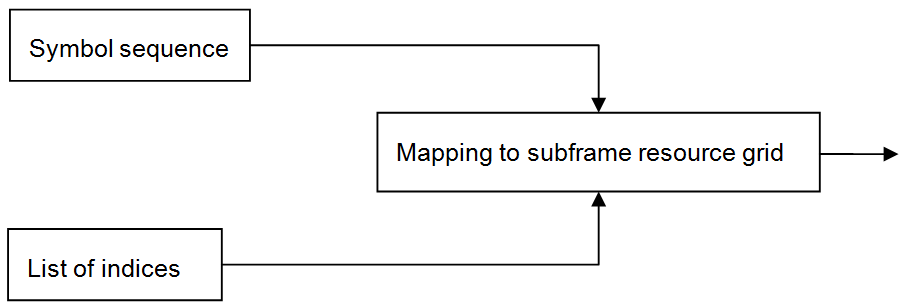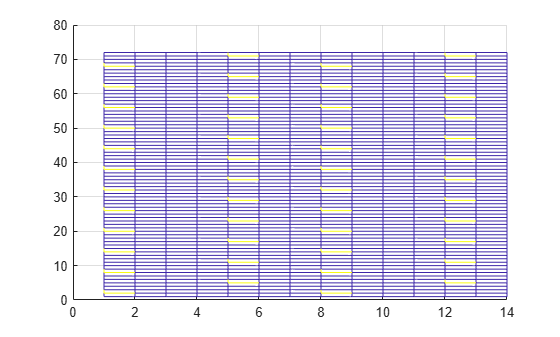Documentation

## Resource Grid Indexing

### Overview

The LTE Toolbox™ provides facilities to generate sequences of symbols corresponding to the physical channels and signals. Indices for the mapping of these sequences to the resource grid are also generated. For convenience, the LTE Toolbox uses the MATLAB® linear indexing style to represent these indices.### Subframe Resource Grid Size

Before applying OFDM modulation (IFFT), the physical channels and signals in LTE are mapped to different portions of the subframe resource grid. The subframe resource grid is represented in the LTE Toolbox as a multidimensional array of the following size.

`$12{N}_{RB}×2{N}_{symb}×P$`

In the preceding expression, ${N}_{RB}$ is the number of resource blocks spanning the available bandwidth, ${N}_{symb}$ is the number of OFDM (or SC-FDMA in the uplink) symbols per slot, and $P$ is the number of antenna ports. Therefore, the resource grid represents a subframe (two slots) and whole bandwidth, since there are 12 subcarriers per resource block. For the single antenna case, a resource grid can be a two-dimensional matrix of the following size.

`$12{N}_{RB}×2{N}_{symb}$`

### Creating an Empty Resource Grid

Create an empty downlink resource grid using two different methods. Valid and equivalent subframe resource grids can be created using the `lteDLResourceGrid` function or the MATLAB® `zeros` function.

Initialize required parameters

Create the parameter structure for normal cyclic prefix, nine downlink resource blocks, and one transmit antenna. Also define seven symbols per slot for use in the `zeros` function only.

```enb.CyclicPrefix = 'Normal'; enb.NDLRB = 9; enb.CellRefP = 1; noSymbolsSlot = 7;```

Create two empty resource grids

Create an empty subframe resource grid, using each method.

```resourceGrid1 = lteDLResourceGrid(enb); resourceGrid2 = zeros(enb.NDLRB*12, noSymbolsSlot*2, enb.CellRefP);```

Confirm the grids are equal

Compare the two grid variables for equality using the MATLAB `isequal` function.

`isequal(resourceGrid1,resourceGrid2)`
```ans = logical 1 ```

Both approaches generate the same result. Use either approach to create an empty downlink resource grid. Similarly, an empty uplink resource grid could be created using `lteULResourceGrid` function or the MATLAB `zeros` function.

### Resource Grid Indexing

Generate a reference signal and map it to an empty resource grid for the single antenna case. The LTE Toolbox(TM) has been designed to facilitate the mapping of physical channels and signals in the resource grid.

Set up the cell-wide settings. Create a structure and specify the cell-wide settings as its fields.

```enb.CyclicPrefix = 'Normal'; enb.NDLRB = 6; enb.CellRefP = 1; enb.NCellID = 1; enb.NSubframe = 0; enb.DuplexMode = 'FDD'; antPort = 0;```

The `enb` structure now contains the parameters required by the functions to be called next.

Use `lteDLResourceGrid` to create an empty subframe resource grid, then populate it with reference symbols. To do so, call the `lteCellRSIndices` and `lteCellRS` functions.

```resourceGrid = lteDLResourceGrid(enb); ind = lteCellRSIndices(enb,antPort); rs = lteCellRS(enb,antPort); resourceGrid (ind) = rs;```

A call to the function `lteCellRSIndices` generates a list of indices identifying to where the reference signal is to be mapped, whereas the call to `lteCellRS` generates the reference signal symbols.

### Linear Indices and Subscripts

Generate indices in linear and subscript form. All of the LTE Toolbox� index generation functions can produce either linear or subscript formats by setting the appropriate options string. The default is linear indexing style, which allows access to any element of a matrix with a single index value. Subscripted matrix element indexing is also available. Using subscripted indexing on a 2-D matrix, you can access each element with a set of two elements representing the row and column equivalents.

Note, the linear indexing style allows you to conveniently map the reference sequence symbols to the appropriate location in the resource grid with just one line of code. Mapping reference symbols to the resource grid using subscripted indices would require more finesse.

Create a structure specifying the cell-wide settings as its fields. Assign zero as the antenna port number.

```enb.CyclicPrefix = 'Normal'; enb.NDLRB = 6; enb.CellRefP = 1; enb.NCellID = 1; enb.NSubframe = 0; enb.DuplexMode = 'FDD'; antPort = 0;```

The `enb` structure now contains the parameters required by the functions to be called next.

Use `lteDLResourceGrid` to create an empty subframe resource grid and `lteCellRS` to create reference signal symbols. View the empty resource grid.

```resourceGrid = lteDLResourceGrid(enb); rs = lteCellRS(enb,antPort); mesh(abs(resourceGrid)) view(2)```After the indices are generated and the reference symbols are mapped to the resource grid, the resource grid is replotted to show the reference symbol mapping.

Generate linear indices. Since linear indexing is the default, the following two calls are equivalent.

```ind_lin = lteCellRSIndices(enb,antPort); ind_lin = lteCellRSIndices(enb,antPort,'ind')```
```ind_lin = 48x1 uint32 column vector 2 8 14 20 26 32 38 44 50 56 ⋮ ```

Mapping the reference signal symbols to the resource grid using linear indices, `ind_lin`, simply requires you to assign the reference signal symbols to the resource grid indicating the active indices.

`resourceGrid(ind_lin) = rs;`

Replotting the resource grid shows the reference symbols active for the cell-wide settings assigned in `enb`.

```figure mesh(abs(resourceGrid)) view(2)```Alternatively, generate indices in subscript form by providing the `'sub'` option string for `lteCellRSIndices`.

`ind_sub = lteCellRSIndices(enb,antPort,'sub')`
```ind_sub = 48x3 uint32 matrix 2 1 1 8 1 1 14 1 1 20 1 1 26 1 1 32 1 1 38 1 1 44 1 1 50 1 1 56 1 1 ⋮ ```

In this case, the output argument, `ind_sub`, is a [subcarrier, OFDM symbol, antenna port] matrix format of the resource grid. These indices are calculated for antenna port 0.

### Converting Between Linear Indices and Subscripts

Conversion between linear indices and subscripts can be achieved using the MATLAB `ind2sub` and `sub2ind` functions. Alternatively, all index generation functions in the LTE Toolbox can produce both formats.

### Multi-Antenna Linear Indices

Generate indices in multi-antenna linear form. This form is a variant of the MATLAB® linear indexing style in which the indices corresponding for each antenna port are in a different column. However, all indices are still in linear form. Several toolbox functions return indices in multi-antenna linear form.

To illustrate this, call the function `ltePDSCH` for the four antenna case.

```enb.CellRefP = 4; enb.CFI = 1; enb.NCellID = 1; enb.NSubframe = 0; enb.NDLRB = 6; enb.CyclicPrefix = 'Normal'; enb.DuplexMode = 'FDD'; pdsch.TxScheme = 'TxDiversity'; pdsch.Modulation = 'QPSK'; pdsch.RNTI = 1; pdsch.PRBSet = (0:5).'; data = ones(768,1); symb = ltePDSCH(enb,pdsch,data); size(symb)```
```ans = 1×2 384 4 ```
`symb(1:10,:)`
```ans = 10×4 complex -0.5000 - 0.5000i 0.0000 + 0.0000i -0.5000 - 0.5000i 0.0000 + 0.0000i 0.5000 - 0.5000i 0.0000 + 0.0000i -0.5000 + 0.5000i 0.0000 + 0.0000i 0.0000 + 0.0000i -0.5000 - 0.5000i 0.0000 + 0.0000i 0.5000 - 0.5000i 0.0000 + 0.0000i -0.5000 - 0.5000i 0.0000 + 0.0000i -0.5000 + 0.5000i 0.5000 - 0.5000i 0.0000 + 0.0000i 0.5000 + 0.5000i 0.0000 + 0.0000i -0.5000 + 0.5000i 0.0000 + 0.0000i 0.5000 + 0.5000i 0.0000 + 0.0000i 0.0000 + 0.0000i 0.5000 + 0.5000i 0.0000 + 0.0000i -0.5000 + 0.5000i 0.0000 + 0.0000i 0.5000 + 0.5000i 0.0000 + 0.0000i 0.5000 - 0.5000i -0.5000 - 0.5000i 0.0000 + 0.0000i 0.5000 + 0.5000i 0.0000 + 0.0000i -0.5000 + 0.5000i 0.0000 + 0.0000i -0.5000 + 0.5000i 0.0000 + 0.0000i ```

The output argument, `symb`, is a matrix with four columns, in which each column corresponds to each antenna port.

In a similar format, generate the indices for the PDSCH by calling `ltePDSCHIndices`.

```pdschIndices = ltePDSCHIndices(enb,pdsch,pdsch.PRBSet); size(pdschIndices)```
```ans = 1×2 384 4 ```
`pdschIndices(1:10,:)`
```ans = 10x4 uint32 matrix 145 1153 2161 3169 146 1154 2162 3170 147 1155 2163 3171 148 1156 2164 3172 149 1157 2165 3173 150 1158 2166 3174 151 1159 2167 3175 152 1160 2168 3176 153 1161 2169 3177 154 1162 2170 3178 ```

Again, each column corresponds to each of the four antenna ports. The concatenation of all four columns produces a column vector of indices using the MATLAB linear indexing style.

### Index Base

Generate either zero-based or one-based indices. All mapping operations in the LTE technical specification (TS) documents refer to zero-based indexing. However, MATLAB® indices must be one-based. LTE Toolbox™ generates one-based indices by default, but you can generate zero-based indices by setting the appropriate options string.

Create a cell-wide setting structure and assign an antenna port number.

```enb.NDLRB = 6; enb.NCellID = 1; enb.CyclicPrefix = 'Normal'; enb.DuplexMode = 'FDD'; antPort = 0;```

Since one-based indexing is the default, you can generate one-based indices by specifying the `'1based'` flag or leaving it out.

```ind = lteCellRSIndices(enb,antPort); ind = lteCellRSIndices(enb,antPort,'1based');```

Generate zero-based indices by specifying the `'0based'` flag.

`ind = lteCellRSIndices(enb,antPort,'0based');`

### Resource Blocks

The 3GPP documents describes a resource block to be a group of resource elements spanning 12 consecutive subcarriers in the frequency domain and one slot in the time domain. For processing efficiency, the LTE Toolbox™, operates on a subframe (two timeslot) basis and describes a resource block pair to represent 12 consecutive subcarriers spanning in the frequency domain and one subframe (two slots) in the time domain. For example, the command `ltePDSCHIndices` uses the parameter `PRBSet` to define the set of physical resource block (PRB) indices for a subframe of data.

Create the cell-wide settings structure and define the `PDSCH` configuration.

```enb.CellRefP = 4; enb.CFI = 1; enb.NCellID = 1; enb.NSubframe = 0; enb.NDLRB = 6; enb.CyclicPrefix = 'Normal'; enb.DuplexMode = 'FDD'; pdsch.TxScheme = 'TxDiversity'; pdsch.Modulation = 'QPSK'; pdsch.RNTI = 1; pdsch.PRBSet = (0:5).';```

Create a set of PDSCH PRB indices for the initialized configuration.

```pdschIndices = ltePDSCHIndices(enb,pdsch,pdsch.PRBSet); size(pdschIndices)```
```ans = 1×2 384 4 ```
`pdschIndices(1:10,:)`
```ans = 10x4 uint32 matrix 145 1153 2161 3169 146 1154 2162 3170 147 1155 2163 3171 148 1156 2164 3172 149 1157 2165 3173 150 1158 2166 3174 151 1159 2167 3175 152 1160 2168 3176 153 1161 2169 3177 154 1162 2170 3178 ```
`pdsch`
```pdsch = struct with fields: TxScheme: 'TxDiversity' Modulation: 'QPSK' RNTI: 1 PRBSet: [6x1 double] ```

`pdsch.PRBSet` can be either a column vector or a two-column matrix. If you provide a column vector, the resource allocation is the same in both slots of the subframe, which means the set of resource indices applies to both subframe time slots. On the other hand, if you provide a two-column matrix, the PRB indices refer to each slot individually.